# How To Make : A Machine Learning Based Sudoku Solving Robot

## !!Attention: Before you start reading this article, please watch the above video once as it is how the final model will work.

Solving Sudoku is not a very easy job for everyone. It may take a few hours for a beginner to solve a simple sudoku puzzle. That’s why we thought if it’s possible to make a robot which can solve any sudoku puzzle(does not matter how much hard it is) by just seeing it just like a normal human. Here we will see how we can make a simple sudoku solver robot which can solve any sudoku puzzle for us within a very few seconds.

## Rules Of Sudoku:

So before we make our own robot to solve sudoku, lets review the rules of sudoku: Sudoku is played on a grid of 9 x 9 spaces. Within the rows and columns are 9 “squares” (made up of 3 x 3 spaces). Each row, column and square (9 spaces each) needs to be filled out with the numbers 1-9, without repeating any numbers within the row, column or square. For example: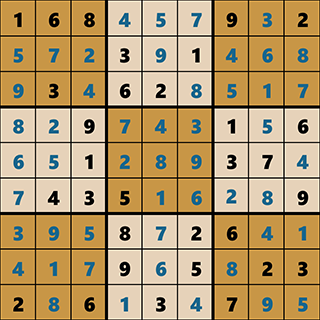In the above image, it’s a solved soduko puzzle. As you can see numbers 1-9 are not repeating within any row, column or square.

## Problem Statement:

Before solving any problem, we must understand the problem and its input-output. In this case, our input will be an image of a sudoku puzzle printed in any white paper which will be captured by our robot. It can be an image of a newspaper which consists of a sudoku puzzle. For eaxmple: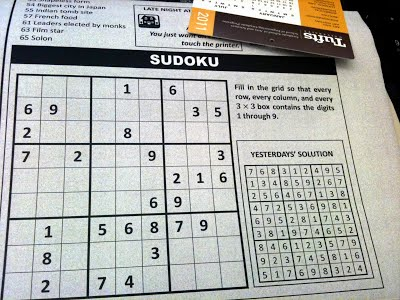The ouput will be the solved puzzle which will be shown in the screen of our robot.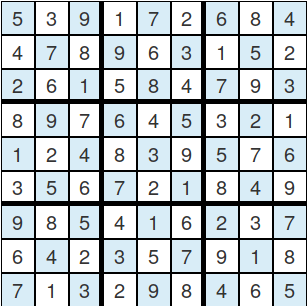## Required Components:

Now at first, we need to design the hardware for the robot. Basically, we need a microprocessor with at least 512 MB ram, a camera module which supports that microprocessor, a screen, and few other accessories. Here we are using the following hardware parts:

1. Raspberry pi 3 B+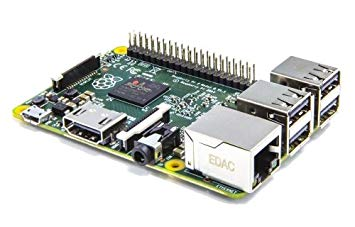2. Raspberry Pi Camera Module V2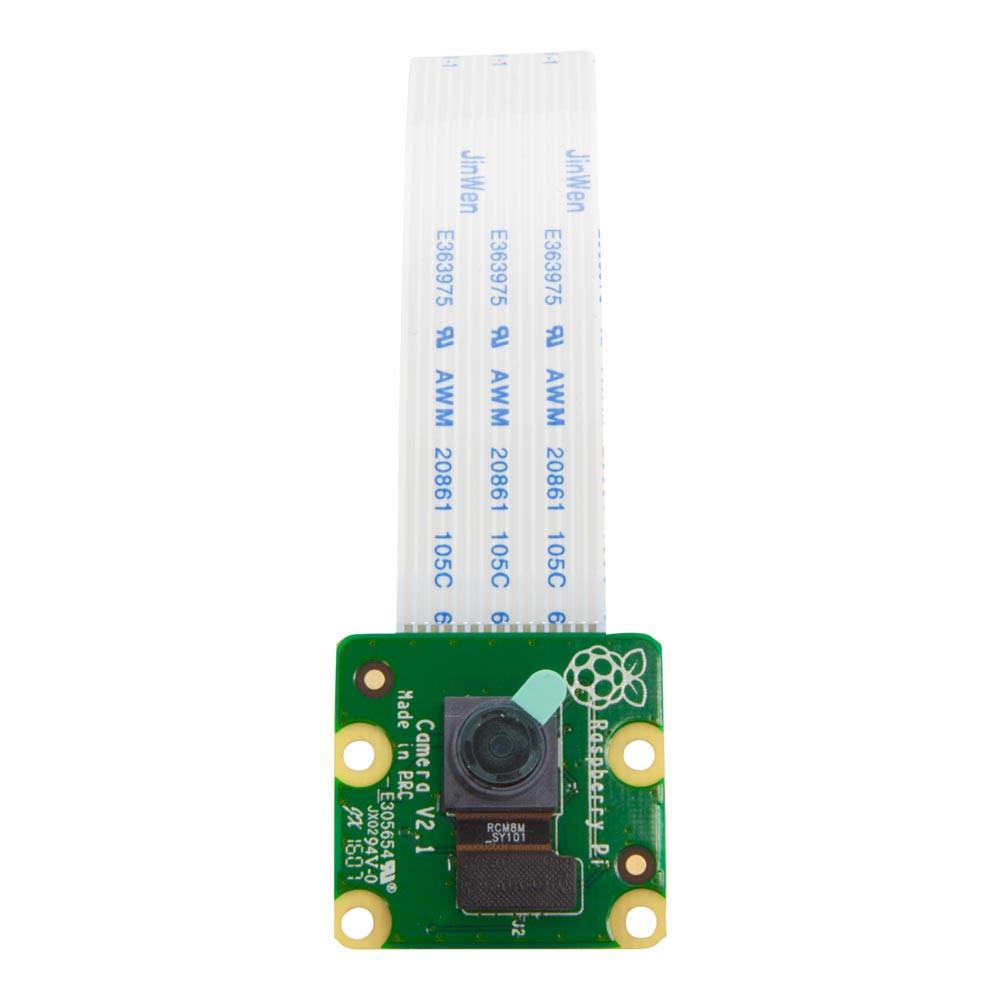3. Raspberry pi Touch Screen Module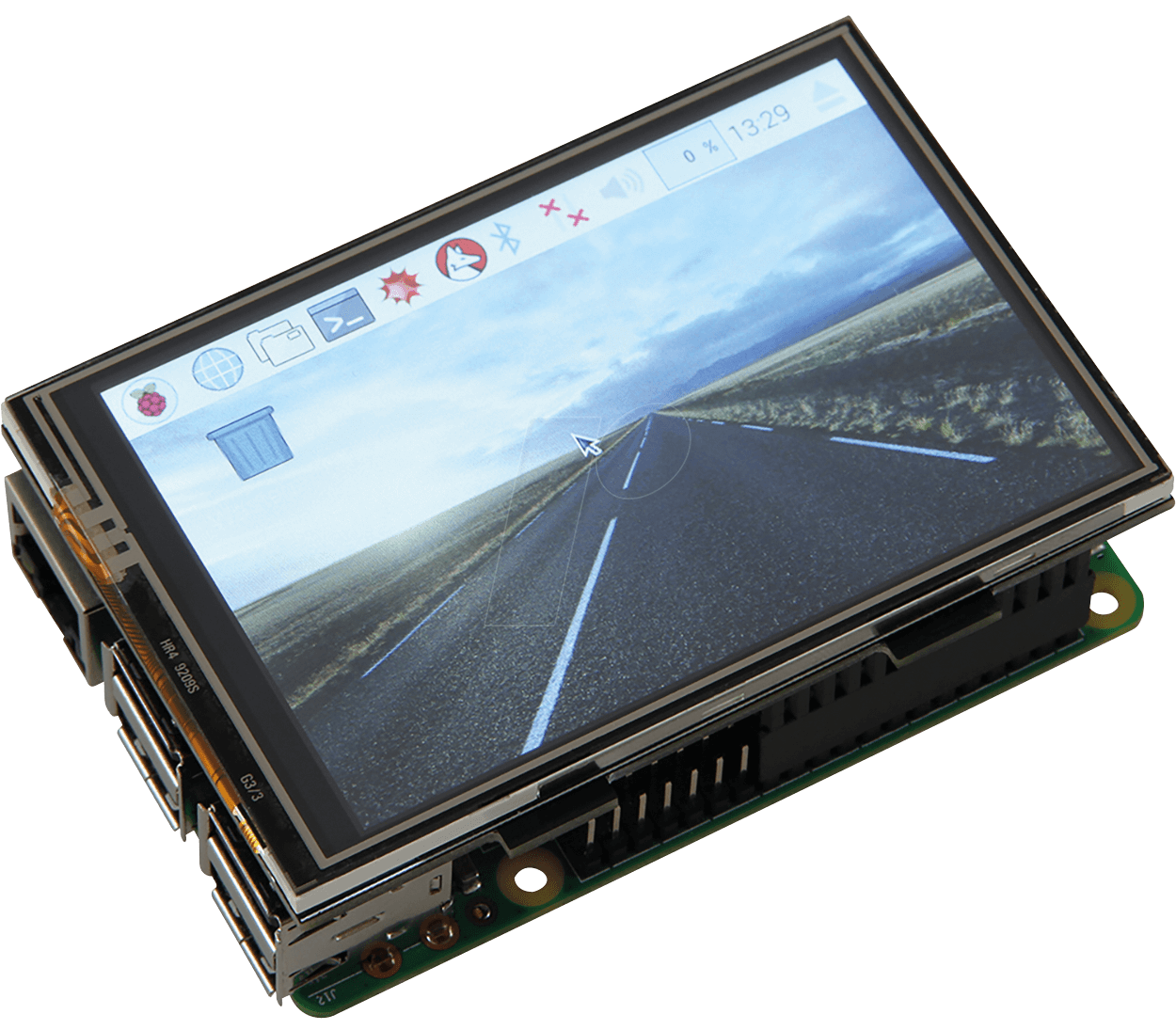4. speaker (small in size)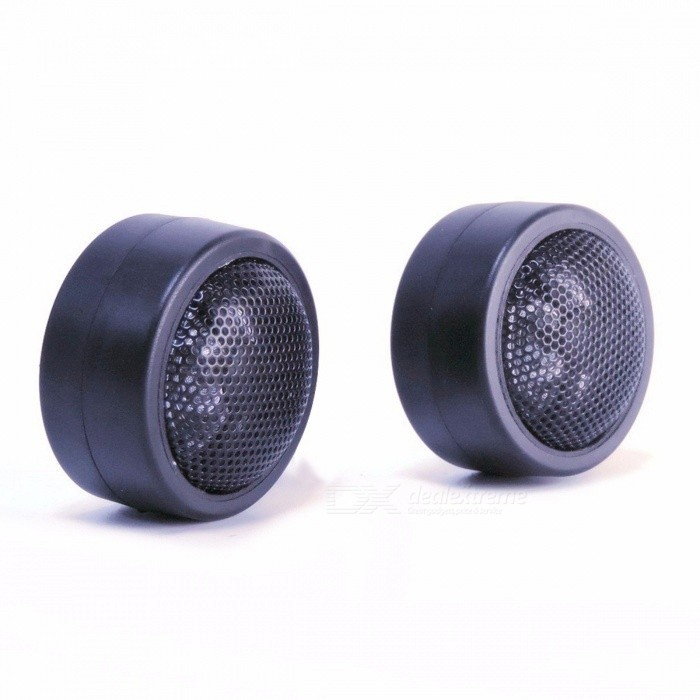5. Led lights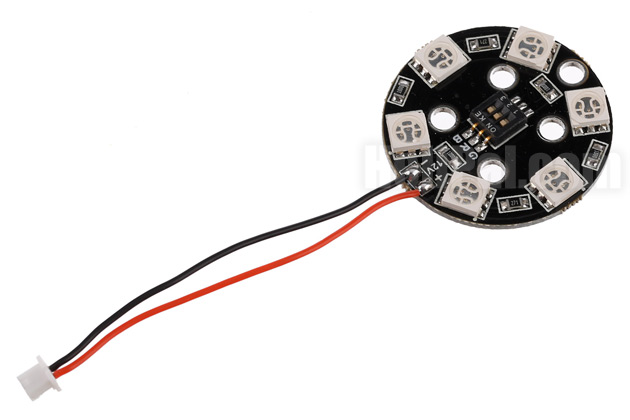6. power supply (2 Amp)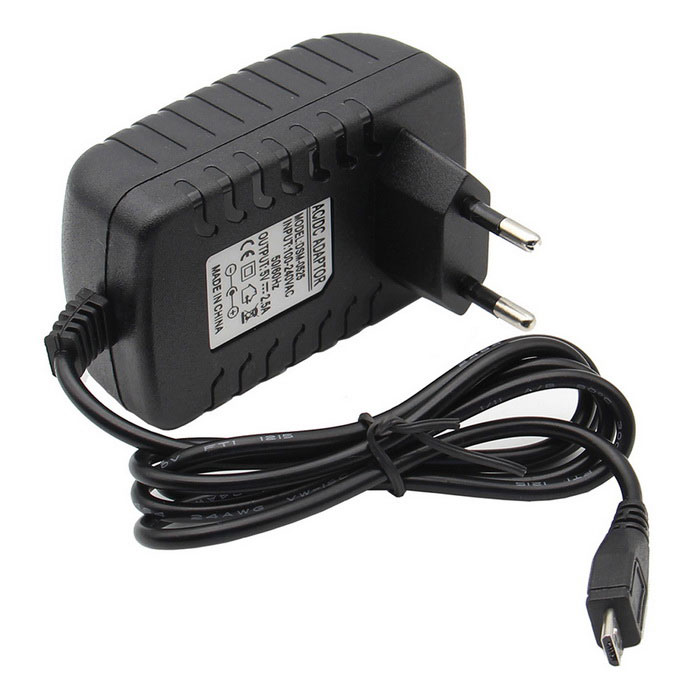## Connecting the Components:

Now to make our hardware ready for programming, we just need to follow the following steps:

1. Install the lastest Rasbian OS in a memory card. For details visit www.raspberrypi.org/documentation/installation/installing-images/.
2. Insert the memory card into raspberry pi.
3. Connect the raspberry pi with the camera module.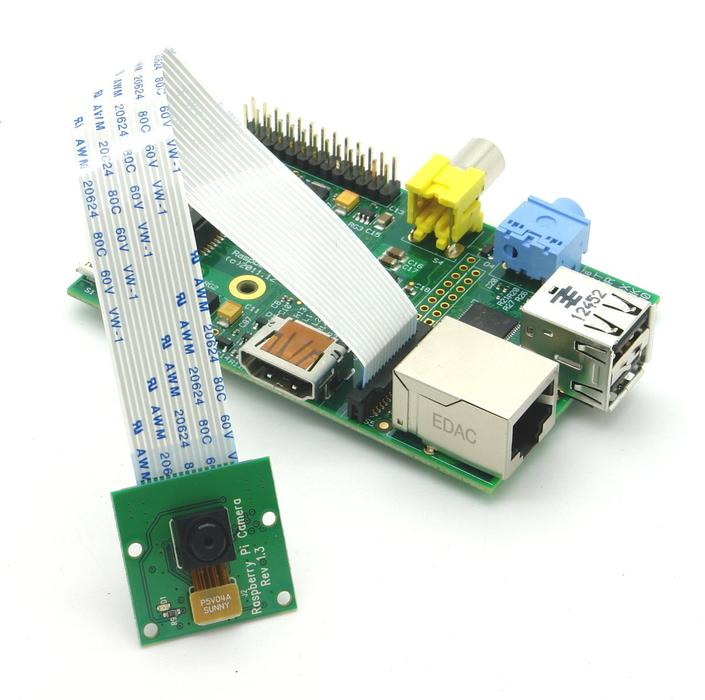4. Connect the speaker through the audio output jack.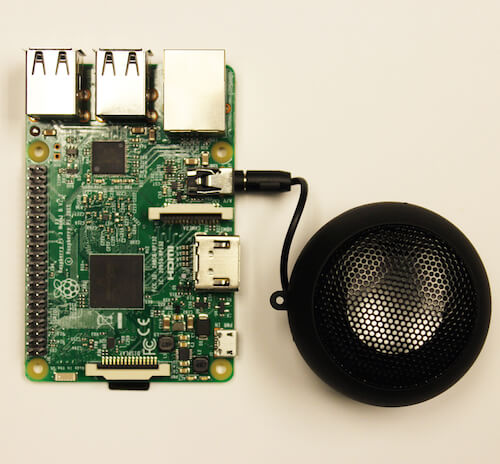5. connect the LEDS with any of the GPIO pins of raspberry pi.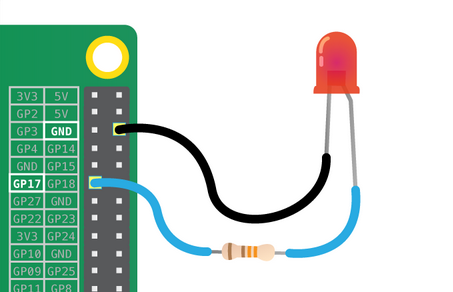6. Conncet the touch screen with raspberry pi.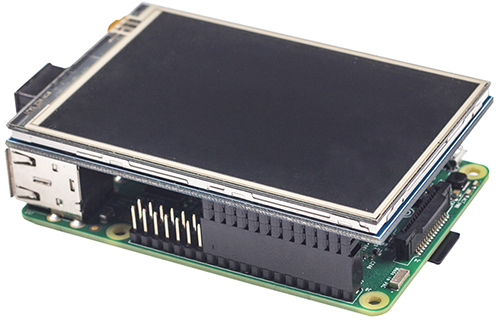7. Power the pi through the adapter.

## Set Up Raspberry Pi for Coding:

Now for programming, we can connect the raspberry pi with a large screen through HDMI, or we can simply use SSH. Before doing any further things, first we need to install the required drivers for the raspberry pi screen (details will be available in the “Touch screen” manual). Also, we need to set up the wifi and other settings.

## Finding a Suitable Body:

Finally we need to put all these components in a single body so that it looks nice. You may 3D print a custom robot body or may search for any ready-made one. Here I have used a broken toy for this purpose. And the end result looks like this: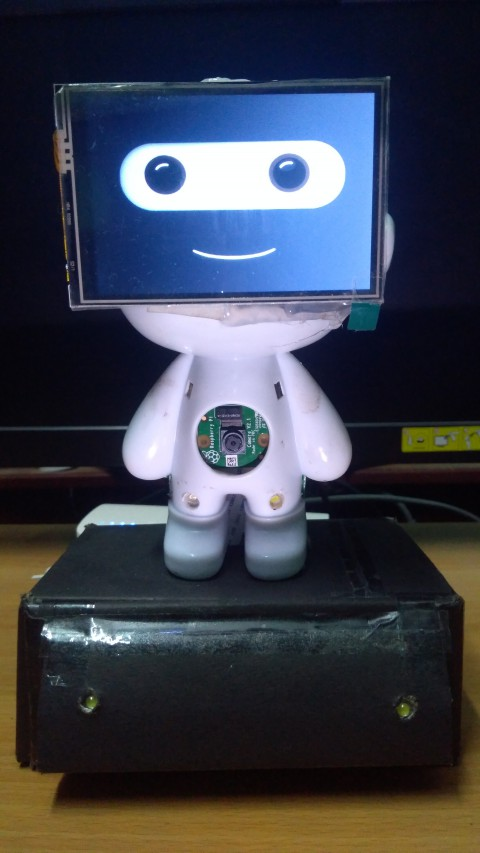Finally our hardware part is ready and now we can go for the coding part.

## Solving the Sudoku:

Now our robot needs some kind of algorithm to solve sudoku puzzles. Here we will use backtracking as it is very much efficient for this kind of applications. Now a simple backtracking algorithm to solve a sudoku looks like this:

``````Find row, col of an unassigned cell
If there is none, return true
For digits from 1 to 9
a) If there is no conflict for digit at row, col
assign digit to row, col and recursively try fill in rest of grid
b) If recursion successful, return true
c) Else, remove digit and try another
If all digits have been tried and nothing worked, return false
source: Geeksforgeeks
``````

One can easily implement this algorithm in any programming language. We have used python 2.7 in this case. The code is shown below:

``````class solver:

def __init__(self,grid):
self.grid=grid
self.digits = '123456789'
self.rows = 'ABCDEFGHI'
self.cols = self.digits
self.squares = self.cross(self.rows, self.cols)
self.unitlist = ([self.cross(self.rows, c) for c in self.cols] +
[self.cross(r, self.cols) for r in self.rows] +
[self.cross(rs, cs) for rs in ('ABC', 'DEF', 'GHI') for cs in ('123', '456', '789')])
self.units = dict((s, [u for u in self.unitlist if s in u])
for s in self.squares)
self.peers = dict((s, set(sum(self.units[s], [])) - set([s]))
for s in self.squares)
self.seq=""
def cross(self,A, B):
"Cross product of elements in A and elements in B."
return [a+b for a in A for b in B]

def test(self):
"A set of unit tests."
assert len(self.squares) == 81
assert len(self.unitlist) == 27
assert all(len(self.units[s]) == 3 for s in self.squares)
assert all(len(self.peers[s]) == 20 for s in self.squares)
assert self.units['C2'] == [['A2', 'B2', 'C2', 'D2', 'E2', 'F2', 'G2', 'H2', 'I2'],
['C1', 'C2', 'C3', 'C4', 'C5', 'C6', 'C7', 'C8', 'C9'],
['A1', 'A2', 'A3', 'B1', 'B2', 'B3', 'C1', 'C2', 'C3']]
assert self.peers['C2'] == set(['A2', 'B2', 'D2', 'E2', 'F2', 'G2', 'H2', 'I2',
'C1', 'C3', 'C4', 'C5', 'C6', 'C7', 'C8', 'C9',
'A1', 'A3', 'B1', 'B3'])
print 'All tests pass.'

def parse_grid(self):
"""Convert grid to a dict of possible values, {square: digits}, or
return False if a contradiction is detected."""
## To start, every square can be any digit; then assign values from the grid.
values = dict((s, self.digits) for s in self.squares)
for s,d in self.grid_values().items():
if d in self.digits and not self.assign(values, s, d):
return False ## (Fail if we can't assign d to square s.)
return values

def grid_values(self):
"Convert grid into a dict of {square: char} with '0' or '.' for empties."
chars = [c for c in self.grid if c in self.digits or c in '0.']
assert len(chars) == 81
return dict(zip(self.squares, chars))

def assign(self,values, s, d):
"""Eliminate all the other values (except d) from values[s] and propagate.
Return values, except return False if a contradiction is detected."""
other_values = values[s].replace(d, '')
if all(self.eliminate(values, s, d2) for d2 in other_values):
return values
else:
return False

def eliminate(self,values, s, d):
"""Eliminate d from values[s]; propagate when values or places <= 2.
Return values, except return False if a contradiction is detected."""
if d not in values[s]:
values[s] = values[s].replace(d,'')
## (1) If a square s is reduced to one value d2, then eliminate d2 from the peers.
if len(values[s]) == 0:
return False ## Contradiction: removed last value
elif len(values[s]) == 1:
d2 = values[s]
if not all(self.eliminate(values, s2, d2) for s2 in self.peers[s]):
return False
## (2) If a unit u is reduced to only one place for a value d, then put it there.
for u in self.units[s]:
dplaces = [s for s in u if d in values[s]]
if len(dplaces) == 0:
return False ## Contradiction: no place for this value
elif len(dplaces) == 1:
# d can only be in one place in unit; assign it there
if not self.assign(values, dplaces, d):
return False
return values

def display(self,values):
"Display these values as a 2-D grid."
l=[]
for i in  self.squares:
l.append(values[i])
self.seq="".join(l)
width = 1+max(len(values[s]) for s in self.squares)
line = '+'.join(['-'*(width*3)]*3)
for r in self.rows:
print ''.join(values[r+c].center(width)+('|' if c in '36' else '')
for c in self.cols)
if r in 'CF': print line
print

def solve(self): return self.search(self.parse_grid())

def search(self,values):
"Using depth-first search and propagation, try all possible values."
if values is False:
return False ## Failed earlier
if all(len(values[s]) == 1 for s in self.squares):
return values ## Solved!
## Chose the unfilled square s with the fewest possibilities
n,s = min((len(values[s]), s) for s in self.squares if len(values[s]) > 1)
return self.some(self.search(self.assign(values.copy(), s, d))
for d in values[s])

def some(self,seq):
"Return some element of seq that is true."
for e in seq:
if e: return e
return False

def main():
sequence = "530070000600195000098000060800060003400803001700020006060000280000419005000080079"
s=solver(sequence)
s.display(s.solve())
if __name__ == '__main__': main()

``````

I am not going to explain the code as it will take a lot of time. But let’s see how to use this code. So if we have a puzzle like this: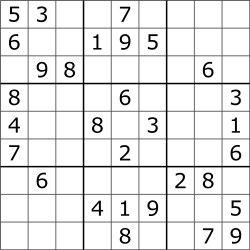We have to write the digits row wise from left to right in sequence. For blanks we have to write ‘0’ in the sequence. So in this case the sequence will be

``````sequence = "530070000600195000098000060800060003400803001700020006060000280000419005000080079"
``````

After this if we execute the program the output will be:

``````5 3 4 |6 7 8 |9 1 2
6 7 2 |1 9 5 |3 4 8
1 9 8 |3 4 2 |5 6 7
------+------+------
8 5 9 |7 6 1 |4 2 3
4 2 6 |8 5 3 |7 9 1
7 1 3 |9 2 4 |8 5 6
------+------+------
9 6 1 |5 3 7 |2 8 4
2 8 7 |4 1 9 |6 3 5
3 4 5 |2 8 6 |1 7 9
``````

As we can see it has solved the sudoku perfectly.

credits : This code is an reimplementation based on the code “Solving Every Sudoku Puzzle” written by Peter Norvig (Director of research at Google). You can visit his site here norvig.com. For the explaination of this code you can visit towardsdatascience.com/peter-norvigs-sudoku-solver-25779bb349ce.

## Recognizing the Sudoku:

Now our robot knows how to solve a sudoku puzzle, but it needs the input. Here comes the little tricky part. It needs to scan an image of a Sudoku puzzle and get the input from that. To do so, we need the help of image processing and machine learning. We will process the captured image and recognize digits from it in a particular sequence. Then we will pass the sequence to the previous sudoku solving program and will get the final output.

Here you can find a very basic tutorial for the image processing part opencvpython.

A special thanks to Mike Deffenbaugh. He has improved that previous code and got it to work pretty well. You can visit his blog here www.mikedeff.in/sudoku.html

But in our case, we need a code for our robot. So all these codes will not work as they were not written for the raspberry pi. So I have modified the code for raspberry pi. Actually, I was in a little hurry, so may be there were some parts of the code which can be much better. So I am leaving that part up to you people. And I also hope that someone will improve the accuracy of this image recognizing part as it can be improved. Here I am writing the modified code for raspberry pi:

``````import cv2
import numpy as np
import sys
import time
import math
import random as rn
import copy
from picamera.array import PiRGBArray
from picamera import PiCamera
from sudoku_solve import solver
import os
import led

def speak(current):
p = current.reshape(1,81)
for i in p:
for j in i:
os.system("espeak  -w out.wav {0} && aplay out.wav".format(j))
class puzzleStatusClass:
# this class defines the actual mathematical properties of the sudoku puzzle
# and the associated methods used to solve the actual sudoku puzzle
def __init__(self):
# .current is a 9x9 grid of all solved values for the puzzle
self.current = np.zeros((9, 9), np.uint8)
self.currentBackup = np.zeros((9, 9), np.uint8)
# .last is used to compare to .current to evaluate whether two consecutive OCR results match
self.last = np.zeros((9, 9), np.uint8)
# .orig is used to store the state of .current that is obtained from OCR,
# but before solving for any new values
self.orig = np.zeros((9, 9), np.uint8)
# .solve starts off by containing 1-9 in a 9 by 9 grid,
# by process of elimination .solve will produce the final solution
self.solve = [[[1, 2, 3, 4, 5, 6, 7, 8, 9] for x in range(9)] for y in range(9)]
self.solveBackup = []
# .change is True when the solver algorithm has made a change to .solve
self.change = True
# .guess is True when the solver has given up on analytical techniques
# and has begun randomly guessing at the solution
self.guess = False

def checkSolution(self):
# check puzzle using three main rules
err = 0  # error code
# 1) no number shall appear more than once in a row
for x in range(9):  # for each row
# count how many of each number exists
check = np.bincount(self.current[x, :])
for i in range(len(check)):
if i == 0:
if check[i] != 0:
err = 1  # incomplete, when the puzzle is complete no zeros should exist
else:
if check[i] > 1:
err = -1  # incorrect, there can't be more than one of any number
print "ERROR in row ", x, " with ", i
return err
# 2) no number shall appear more than once in a column
for y in range(9):  # for each column
check = np.bincount(self.current[:, y])
for i in range(len(check)):
if i == 0:
if check[i] != 0:
err = 1  # incomplete
else:
if check[i] > 1:
err = -1  # incorrect
print "ERROR in col ", y, " with ", i
return err
# 3) no number shall appear more than once in a 3x3 cell
for x in range(3):
for y in range(3):
check = np.bincount(self.current[x * 3:x * 3 + 3, y * 3:y * 3 + 3].flatten())
for i in range(len(check)):
if i == 0:
if check[i] != 0:
err = 1  # incomplete
else:
if check[i] > 1:
err = -1  # incorrect
print "ERROR in box ", x, y, " with ", i
return err
return err

class imageClass:
#this class defines all of the important image matrices, and information about the images.
#also the methods associated with capturing input, displaying the output,
#and warping and transforming any of the images to assist with OCR
def __init__(self):
#.captured is the initially captured image
self.captured = []
#.gray is the grayscale captured image
self.gray = []
#.thres is after adaptive thresholding is applied
self.thresh = []
#.contours contains information about the contours found in the image
self.contours = []
#.biggest contains a set of four coordinate points describing the
#contours of the biggest rectangular contour found
self.biggest = None;
#.maxArea is the area of this biggest rectangular found
self.maxArea = 0
#.output is an image resulting from the warp() method
self.output = []
self.outputBackup = []
self.outputGray = []
#.mat is a matrix of 100 points found using a simple gridding algorithm
#based on the four corner points from .biggest
self.mat = np.zeros((100,2),np.float32)
#.reshape is a reshaping of .mat
self.reshape = np.zeros((100,2),np.float32)

def captureImage(self,status):
#captures the image and finds the biggest rectangle
camera = PiCamera()
camera.resolution = (480, 400)
camera.framerate = 32
rawCapture = PiRGBArray(camera, size=(480, 400))

# allow the camera to warmup
time.sleep(0.1)
#try:
for frame in camera.capture_continuous(rawCapture, format="bgr", use_video_port=True):
#rgb, _ = freenect.sync_get_video()
#cv2.namedWindow("SUDOKU Solver")
#vc = cv2.VideoCapture(1)
#if vc.isOpened():  # try to get the first frame
#else:
#   rval = False
#bgr = cv2.cvtColor(rgb, cv2.COLOR_BGR2R
image = frame.array
#cv2.imshow("Frame", image)
#key = cv2.waitKey(1) & 0xFF
self.captured = image
rawCapture.truncate(0)
#except TypeError:
#print "No Kinect Detected!"
# for testing purposes
#self.captured = cv2.resize(img, (600, 600))

# convert to grayscale
self.gray = cv2.cvtColor(self.captured, cv2.COLOR_BGR2GRAY)
print "gray"
# noise removal with gaussian blur
self.gray = cv2.GaussianBlur(self.gray, (5, 5), 0)
self.thresh = cv2.adaptiveThreshold(self.gray, 255, 1, 1, 11, 2)

# find countours in threshold image
self.contours, hierarchy = cv2.findContours(self.thresh, cv2.RETR_TREE, cv2.CHAIN_APPROX_SIMPLE)

# evaluate all blobs to find blob with biggest area
# biggest rectangle in the image must be sudoku square
self.biggest = None
self.maxArea = 0
for i in self.contours:
area = cv2.contourArea(i)
if area > 50000:  # 50000 is an estimated value for the kind of blob we want to evaluate
peri = cv2.arcLength(i, True)   #return the perimeter of the contour
approx = cv2.approxPolyDP(i, 0.02 * peri, True)
if area > self.maxArea and len(approx) == 4:
self.biggest = approx
self.maxArea = area
best_cont = i
if status.detect == 20:
led.turn_on()
if status.detect == 25:
status.puzzleFound = True
print "Sudoku puzzle detected!"
if self.maxArea > 0:
status.noDetect = 0  # reset
status.detect += 1
# draw self.biggest approx contour
if status.completed:
cv2.polylines(self.captured, [self.biggest], True, (0, 255, 0), 3)
elif status.puzzleFound:
cv2.polylines(self.captured, [self.biggest], True, (0, 255, 255), 3)
else:
cv2.polylines(self.captured, [self.biggest], True, (0, 0, 255), 3)
self.reorder()  # reorder self.biggest
else:
status.noDetect += 1
if status.noDetect == 20:
led.turn_off()
print "No sudoku puzzle detected!"
if status.noDetect > 50:
#status.restart = True
pass
"""if status.detect == 25:
status.puzzleFound = True
print "Sudoku puzzle detected!"""
if status.beginSolver == False or self.maxArea == 0:
cv2.imshow('sudoku', self.captured)
key = cv2.waitKey(10)
if key == 27:
sys.exit()
if status.puzzleFound == True:
camera.close()
break

def reorder(self):
#reorders the points obtained from finding the biggest rectangle
#[top-left, top-right, bottom-right, bottom-left]
a = self.biggest.reshape((4,2))
b = np.zeros((4,2),dtype = np.float32)

diff = np.diff(a,axis = 1) #y-x
b = a[np.argmin(diff)] #min diff
b = a[np.argmax(diff)] #max diff
self.biggest = b

def perspective(self):
#create 100 points using "biggest" and simple gridding algorithm,
#these 100 points define the grid of the sudoku puzzle
#topLeft-topRight-bottomRight-bottomLeft = "biggest"
b = np.zeros((100,2),dtype = np.float32)
c_sqrt=10
if self.biggest.any == None:
self.biggest = [[0,0],[640,0],[640,480],[0,480]]
tl,tr,br,bl = self.biggest,self.biggest,self.biggest,self.biggest
for k in range (0,100):
i = k%c_sqrt
j = k/c_sqrt
ml = [tl+(bl-tl)/9*j,tl+(bl-tl)/9*j]
mr = [tr+(br-tr)/9*j,tr+(br-tr)/9*j]
##            self.mat[k,0] = ml+(mr-ml)/9*i
##            self.mat[k,1] = ml+(mr-ml)/9*i
self.mat.itemset((k,0),ml+(mr-ml)/9*i)
self.mat.itemset((k,1),ml+(mr-ml)/9*i)
self.reshape = self.mat.reshape((c_sqrt,c_sqrt,2))

def warp(self):
#take distorted image and warp to flat square for clear OCR reading
kernel = cv2.getStructuringElement(cv2.MORPH_ELLIPSE,(11,11))
close = cv2.morphologyEx(self.gray,cv2.MORPH_CLOSE,kernel)
division = np.float32(self.gray)/(close)
result = np.uint8(cv2.normalize(division,division,0,255,cv2.NORM_MINMAX))
result = cv2.cvtColor(result,cv2.COLOR_GRAY2BGR)
output = np.zeros((450,450,3),np.uint8)
c_sqrt=10
for i,j in enumerate(self.mat):
ri = i/c_sqrt
ci = i%c_sqrt
if ci != c_sqrt-1 and ri != c_sqrt-1:
source = self.reshape[ri:ri+2, ci:ci+2 , :].reshape((4,2))
dest = np.array( [ [ci*450/(c_sqrt-1),ri*450/(c_sqrt-1)],[(ci+1)*450/(c_sqrt-1),
ri*450/(c_sqrt-1)],[ci*450/(c_sqrt-1),(ri+1)*450/(c_sqrt-1)],
[(ci+1)*450/(c_sqrt-1),(ri+1)*450/(c_sqrt-1)] ], np.float32)
trans = cv2.getPerspectiveTransform(source,dest)
warp = cv2.warpPerspective(result,trans,(450,450))
output[ri*450/(c_sqrt-1):(ri+1)*450/(c_sqrt-1) , ci*450/(c_sqrt-1):(ci+1)*450/
(c_sqrt-1)] = warp[ri*450/(c_sqrt-1):(ri+1)*450/(c_sqrt-1) ,
ci*450/(c_sqrt-1):(ci+1)*450/(c_sqrt-1)].copy()
output_backup = np.copy(output)
cv2.imshow('output',output)
key = cv2.waitKey(1)
self.output = output
self.outputBackup = output_backup

def virtualImage(self, puzzle,status):
# output known sudoku values to the real image
j = 0
tsize = (math.sqrt(self.maxArea)) / 400
w = int(20 * tsize)
h = int(25 * tsize)
for i in range(100):
##            x = int(self.mat[i]+8*tsize)
##            y = int(self.mat[i]+8*tsize)
x = int(self.mat.item(i, 0) + 8 * tsize)
y = int(self.mat.item(i, 1) + 8 * tsize)
if i % 10 != 9 and i / 10 != 9:
yc = j % 9
xc = j / 9
j += 1
if puzzle.original[xc, yc] == 0 and puzzle.current[xc, yc] != 0:
string = str(puzzle.current[xc, yc])
cv2.putText(self.captured, string, (x + w / 4, y + h), 0, tsize, (0, 0, 0), 2)
if status.completed:
#pl=self.path.split('.')
#name=pl+"_solved."+pl
name= "solve"+str(rn.randint(0,5000))+".jpg"
print name
cv2.imwrite(name, self.captured)
led.turn_off()
#os.system("espeak -g 5 -w out.wav 'I have solved the puzzle' && aplay out.wav")
os.system("mplayer solved.mp3")
#t1.start()
cv2.imshow('sudoku', self.captured)
"""p = puzzle.current.reshape(1,81)
for i in p:
for j in i:
os.system("espeak  -w out.wav {0} && aplay out.wav".format(j))"""
key = cv2.waitKey(1000000000)
if key == 27:
#sys.exit()
cv2.destroyAllWindows()
#t1.join()
class OCRmodelClass:
#this class defines the data used for OCR,
#and the associated methods for performing OCR
def __init__(self):
responses = responses.reshape((responses.size,1))
#.model uses kNearest to perform OCR
self.model = cv2.KNearest()
self.model.train(samples,responses)
#.iterations contains information on what type of morphology to use
self.iterations = [-1,0,1,2]
self.lvl = 0 #index of .iterations

def OCR(self,status,image,puzzle):
#preprocessing for OCR
#convert image to grayscale
gray = cv2.cvtColor(image.output, cv2.COLOR_BGR2GRAY)
#noise removal with gaussian blur
gray = cv2.GaussianBlur(gray,(5,5),0)
image.outputGray = gray

#attempt to read the image with 4 different morphology values and find the best result
self.success = [0,0,0,0]
self.errors = [0,0,0,0]
for self.lvl in self.iterations:
image.output = np.copy(image.outputBackup)
if self.errors[self.lvl+1]==0:
self.errors[self.lvl+1] = puzzle.checkSolution()
best = 8
for i in range(4):
if self.success[i] > best and self.errors[i]>=0:
best = self.success[i]
ibest = i
print "success:",self.success
print "errors:",self.errors

if best==8:
print "ERROR - OCR FAILURE"
status.restart = True
else:
print "final morph erode iterations:",self.iterations[ibest]
image.output = np.copy(image.outputBackup)
self.lvl = self.iterations[ibest]
cv2.imshow('output',image.output)
key = cv2.waitKey(1)

#perform actual OCR using kNearest model
if self.lvl >= 0:
morph = cv2.morphologyEx(thresh,cv2.MORPH_ERODE,None,iterations = self.lvl)
elif self.lvl == -1:
morph = cv2.morphologyEx(thresh,cv2.MORPH_DILATE,None,iterations = 1)

thresh_copy = morph.copy()
#thresh2 changes after findContours
contours,hierarchy = cv2.findContours(morph,cv2.RETR_LIST,cv2.CHAIN_APPROX_SIMPLE)
thresh = thresh_copy

puzzle.current = np.zeros((9,9),np.uint8)

# testing section
for cnt in contours:
if cv2.contourArea(cnt)>20:
[x,y,w,h] = cv2.boundingRect(cnt)
if  h>20 and h<40 and w>8 and w<40:
if w<20:
diff = 20-w
x -= diff/2
w += diff
sudox = x/50
sudoy = y/50
cv2.rectangle(image.output,(x,y),(x+w,y+h),(0,0,255),2)
#prepare region of interest for OCR kNearest model
roi = thresh[y:y+h,x:x+w]
roismall = cv2.resize(roi,(25,35))
roismall = roismall.reshape((1,875))
roismall = np.float32(roismall)
#find result
retval, results, neigh_resp, dists = self.model.find_nearest(roismall, k = 1)
if results!=0:
string = str(int((results)))
if puzzle.current[sudoy,sudox]==0:
puzzle.current[sudoy,sudox] = int(string)
else:
self.success[self.lvl+1]+=1
cv2.putText(image.output,string,(x,y+h),0,1.4,(255,0,0),3)
else:

class solverStatusClass:
#this class defines the status of the main loop
def __init__(self):
#.beginSolver becomes true when the puzzle is completely captured and ready to solve
self.beginSolver = False
#.puzzleFound becomes true when the puzzle is thought to be found but not yet read with OCR
self.puzzleFound = False
#.puzzleRead becomes true when OCR has confirmed the puzzle
#.restart becomes true when the main loop needs to restart
self.restart = False
#.completed becomes true when the puzzle has been solved
self.completed = False
#.number of times imageClass.captureImage() detects no puzzle
self.noDetect = 0
#.number of times imageClass.captureImage() detects a puzzle
self.detect = 0

def main():
try:
while True:
speak = 0
#try:
status = solverStatusClass()
while status.beginSolver == False:
#status = solverStatusClass()
puzzle = puzzleStatusClass()
image = imageClass()
print "Waiting for puzzle..."
while status.puzzleFound == False:
image.captureImage(status)
if status.restart == True:
break
while status.puzzleRead == False and status.puzzleFound == True :
if speak != 1:
os.system("espeak -g 5 -w out.wav 'I found a puzzle' && aplay out.wav")
speak = 1
time.sleep(2)
#image.captureImage(status)
image.perspective()
image.warp()
if status.restart == True:
print "Restarting..."
break
elif np.array_equal(puzzle.current, puzzle.last):
status.beginSolver = True
print puzzle.current
puzzle.original = np.copy(puzzle.current)
#image.virtualImage(puzzle)
else:
print "Rechecking for Puzzle Match..."
puzzle.last = np.copy(puzzle.current)

if status.beginSolver == True:
os.system("espeak -g 5 -w out.wav 'I have recognised the puzzle and now I am trying to solve it' && aplay out.wav")
print "solving start"
s=puzzle.original.reshape(1,81)
s=s.astype("S")
l = s.tolist()
p = "".join(l)

sudo_solver = solver(p)
sudo_solver.display(sudo_solver.solve())
status.completed = True

solved_seq = np.fromstring(sudo_solver.seq, np.int8) - 48
solved_seq=solved_seq.reshape(9,9)
puzzle.current = np.copy(solved_seq)
print puzzle.current
"""p = puzzle.current.reshape(1,81)
for i in p:
for j in i:
os.system("espeak  -w out.wav {0} && aplay out.wav".format(j))"""
puzzle.solve = np.copy(solved_seq)
#image.virtualImage(puzzle)
print "puzzle solved"
"""if status.completed:
cv2.polylines(image.captured, [self.biggest], True, (0, 255, 0), 3)
elif status.puzzleFound:
cv2.polylines(image.captured, [self.biggest], True, (0, 255, 255), 3)"""

image.perspective()
image.virtualImage(puzzle,status)

#image.captureImage(status)
#if image.maxArea > 0:
"""except:
print "Error occured, restarting....."
continue"""
except AttributeError:
os.system("espeak -g 5 -w out.wav 'I am unable to recognise it ,please show me again' && aplay out.wav")
main()

except KeyboardInterrupt:
led.turn_off()

if __name__ == '__main__': main()
``````

Here you need to install few python libraries and tools:

1. openCV2 (apt-get install python-opencv)
2. picamera
3. espeak (apt-get install espeak)

Also don’t forget to save the sudoku solver code in the same directory as “sudoku-solve.py”. You will also need some trained files to run this code. You can find all that here www.mikedeff.in/sudoku.html. The credit goes to Mike Deffenbaugh for creating these trained files.

Just download these files and save them in the same folder. Then run the main.py program.

## Showcasing Our Sudoku Solver

So we gave it a name SUDO and showcased it in “Kolkata Mini Makers Faire 2019” (First time in Eastern India). And we got a great appreciation from the audience.

### The Author :## Arijit Das

I am a computer science engineering student. I believe that piece of code is mightier than pen as well as a sword. Programming and Solving problems are my passion and that what I strive to practice for my career.